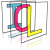Image Component Library (ICL)
icl::cv::HungarianAlgorithm< real > Class Template Reference

Implementation of the Hungarian Algorithm to solve Linear Assignment problems. More...

`#include <HungarianAlgorithm.h>`

## Static Public Member Functions

static std::vector< int > apply (const utils::Array2D< real > &m, bool isCostMatrix=true)
calculate best assignment given cost matrix m More...

static void visualizeAssignment (const utils::Array2D< real > &cost, const std::vector< int > &assignment)
visualized the assignment with given cost matrix and assignment vector More...

## Private Types

typedef utils::Array2D< real > mat
Internal used cost matrix type. More...

## Detailed Description

### template<class real> class icl::cv::HungarianAlgorithm< real >

Implementation of the Hungarian Algorithm to solve Linear Assignment problems.

PositionTracker

# Assignment Problems (LAP)

A LAP is defined as follows: You have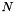workers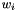andtasks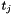. Assigning a certain workerto a certain taskproduces costs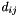which are defined by a square cost matrix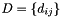. Each worker can only be assigned to perform a single task, and each task has to be processed. The problem is to find the optimal assignment to minimize the arising costs.

# Benchmarks:

• 41ms for a 100² matrix
• 0.0375ms for 10² matrix
• 12s for a 500² matrix

## ◆ mat

template<class real >
 typedef utils::Array2D icl::cv::HungarianAlgorithm< real >::mat
private

Internal used cost matrix type.

## ◆ apply()

template<class real >
 static std::vector icl::cv::HungarianAlgorithm< real >::apply ( const utils::Array2D< real > & m, bool isCostMatrix = `true` )
static

calculate best assignment given cost matrix m

if isCostMatrix is false, its elements are internally multiplied by -1

## ◆ visualizeAssignment()

template<class real >
 static void icl::cv::HungarianAlgorithm< real >::visualizeAssignment ( const utils::Array2D< real > & cost, const std::vector< int > & assignment )
static

visualized the assignment with given cost matrix and assignment vector

The documentation for this class was generated from the following file: